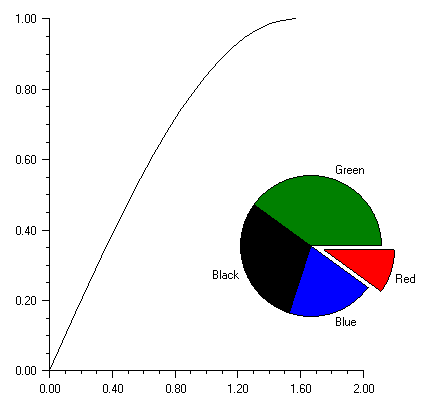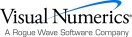# Appendix C: Picture-in-Picture

The picture-in-picture effect can be obtained by using the Viewport attribute. This sets the fraction of the screen into which the chart is to be drawn. The Viewport attribute’s value is a double containing {xmin, xmax, ymin, ymax}, on a [0,1] by [0,1] grid with (0,0) at the top-left.This chart tree for the above picture consists of a chart with an AxisXY child and a Pie child. (The Pie class is a subclass of Axis.) The Viewport attribute of the Pie node is set to a non-default value.

using System;

using System.Windows.Forms;

using System.Drawing;

using Imsl.Chart2D;

public class SamplePnP : FrameChart

{

public SamplePnP()

{

Chart chart = this.Chart;

CreateLineChart(chart);

CreatePieChart(chart);

}

private void CreateLineChart(Chart chart) {

AxisXY axis = new AxisXY(chart);

int npoints = 20;

double dx = 0.5 * Math.PI/(npoints-1);

double[] x = new double[npoints];

double[] y = new double[npoints];

for (int i = 0;  i < x.Length;  i++){

x[i] = i * dx;

y[i] = Math.Sin(x[i]);

}

new Data(axis, x, y);

}

private void CreatePieChart(Chart chart) {

double[] y = new double[] {10, 20, 30, 40};

Pie pie = new Pie(chart, y);

pie.LabelType = Pie.LABEL_TYPE_TITLE;

pie.SetViewport(0.5, 0.9, 0.3, 0.8);

PieSlice[] slice = pie.GetPieSlice();

slice.SetTitle("Red");

slice.FillColor = Color.Red;

slice.Explode = 0.2;

slice.SetTitle("Blue");

slice.FillColor = Color.Blue;

slice.SetTitle("Black");

slice.FillColor = Color.Black;

slice.SetTitle("Green");

slice.FillColor = Color.Green;

}

public static void Main(string[] argv)

{

System.Windows.Forms.Application.Run(new SamplePnP());

}

}Visual Numerics - Developers of IMSL and PV-WAVEhttp://www.vni.com/PHONE: 713.784.3131FAX:713.781.9260